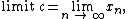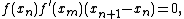# numerical analysis

(redirected from Numerical method)
Also found in: Dictionary, Thesaurus, Acronyms.

## numerical analysis

[nü′mer·i·kəl ə′nal·ə·səs]
(mathematics)
The study of approximation techniques using arithmetic for solutions of mathematical problems.

## Numerical analysis

The development and analysis of computational methods (and ultimately of program packages) for the minimization and the approximation of functions, and for the approximate solution of equations, such as linear or nonlinear (systems of) equations and differential or integral equations. Originally part of every mathematician's work, the subject is now often taught in computer science departments because of the tremendous impact which computers have had on its development. Research focuses mainly on the numerical solution of (nonlinear) partial differential equations and the minimization of functions.

Numerical analysis is needed because answers provided by mathematical analysis are usually symbolic and not numeric; they are often given implicitly only, as the solution of some equation, or they are given by some limit process. A further complication is provided by the rounding error which usually contaminates every step in a calculation (because of the fixed finite number of digits carried).

Even in the absence of rounding error, few numerical answers can be obtained exactly. Among these are (1) the value of a piece-wise rational function at a point and (2) the solution of a (solvable) linear system of equations, both of which can be produced in a finite number of arithmetic steps. Approximate answers to all other problems are obtained by solving the first few in a sequence of such finitely solvable problems. A typical example is provided by Newton's method: A solution c to a nonlinear equation ƒ(c) = 0 is found as thewith Xn+1 being a solution to the linear equationthat is, xn+1 = xn - f(xn)/(xn), n = 0, 1, 2, … Of course, only the first few terms in this sequence x0, x1 x2, … can ever be calculated, and thus one must consider when to break off such a solution process and how to gauge the accuracy of the current approximation.

An otherwise satisfactory computational process may become useless, because of the amplification of rounding errors. A computational process is called stable to the extent that its results are not spoiled by rounding errors. The extended calculations involving millions of arithmetic steps now possible on computers have made the stability of a computational process a prime consideration.

#### Interpolation and approximation

Polynomial interpolation provides a polynomial p of degree n or less which uniquely matches given function values f(x0), …, f(xn) at corresponding distinct points x0, …, xn. The interpolating polynomial p is used in place of f, for example in evaluation, integration, differentiation, and zero finding. Accuracy of the interpolating polynomial depends strongly on the placement of the interpolation points, and usually degrades drastically as one moves away from the interval containing these points (that is, in case of extrapolation).

When many interpolation points (more than 5 or 10) are to be used, it is often much more efficient to use instead a piece-wise polynomial interpolant or spline. Suppose the interpolation points above are ordered, x0 < x1 < ··· xn. Then the cubic spline interpolant to the above data, for example, consists of cubic polynomial pieces, with the ith piece defining the interpolant on the interval [xi-1,xi] and so matched with its neighboring piece or pieces that the resulting function not only matches the given function values (hence is continuous) but also has a continuous first and second derivative.

Interpolation is but one way to determine an approximant. In full generality, approximation involves several choices: (1) a set P of possible approximants, (2) a criterion for selecting from P a particular approximant, and (3) a way to measure the approximation error, that is, the difference between the function ƒ to be approximated and the approximant p, in order to judge the quality of approximation.

#### Solution of linear systems

Solving a linear system of equations is probably the most frequently confronted computational task. It is handled either by a direct method, that is, a method which obtains the exact answer in a finite number of steps, or by an iterative method, or by a judicious combination of both. Analysis of the effectiveness of possible methods has led to a workable basis for selecting the one which best fits a particular situation.

Direct methods require a number of operations which increases with the cube of the number of unknowns. Some types of problems arise wherein the matrix of coefficients is sparse, but the unknowns may number several thousand; for these, direct methods are prohibitive in computer time required. One frequent source of such problems is the finite difference treatment of partial differential equations. A significant literature of iterative methods exploiting the special properties of such equations is available. For certain restricted classes of difference equations, the error in an initial iterate can be guaranteed to be reduced by a fixed factor, using a number of computations that is proportional to n log n, where n is the number of unknowns. Since direct methods require work proportional to n3, it is not surprising that as n becomes large, iterative methods are studied rather closely as practical alternatives.

#### Differential equations

Classical methods yield practical results only for a moderately restricted class of ordinary differential equations, a somewhat more restricted class of systems of ordinary differential equations, and a very small number of partial differential equations. The power of numerical methods is enormous here, for in quite broad classes of practical problems relatively straightforward procedures are guaranteed to yield numerical results, whose quality is predictable.

References in periodicals archive ?
3] Table 5: CPU time for the numerical solution by different methods Numerical method CPU time (sec) Euler 0.
In this section, we'll show the main theoretical results which rules numerical methods of solving differential equations and differential equation systems.
The represented achievements establish better understanding of the meshless numerical methods and broaden their application.
Handbook of Applicable Mathematics: Numerical Methods, Vol.
To find a sufficient condition for an order of a numerical method, we will first consider the behavior of the numerical method for h - 0 and for some fixed N G N (consequently [t.
Thus, in order to verify the order of the error in the numerical method, we graph log E(h) versus log [absolute value of h] for several different values of [absolute value of h], the points {(log [absolute value of h], log [absolute value of E(h)])} should approximately lie on a straight line.
Two numerical methods now routinely being used are linear least-squares fitting and target-factor analysis.
Application and Solution of the Heat Equation in One-and Two-Dimensional Systems Using Numerical Methods University of Tennessee Knoxville TN March 1999.
Moreover, the model and the software used embedded numerical methods, i.
The Method of Lines is a numerical method for solving partial differential equations that describe physical phenomena, and Pregla (Fern U.

Site: Follow: Share:
Open / Close